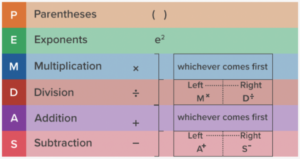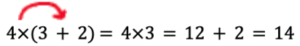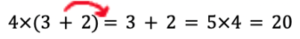# What is the Order of Operations? Definition, Tips & Examples

In maths, the order of operations is a set of rules that helps solve extensive mathematical equations. Understanding the order of operations supports students in answering long, and sometimes algebraic, expressions that can seem daunting for primary school students.

It is important for primary school students to understand the order of operations as they start to learn the basic principles of maths. An easy way to remember the order of operations is through an acronym called PEDMAS.

## What is the Order of Operations?

In simple terms the order of operations is almost like a check list to help students solve equations that involve multiplication, division, addition, and subtraction.

It is vital for students to memorise the order of operations as it is easy to calculate the wrong solution from an equation if the student does not follow the order of operations.

For example, without the order of operations students may be left thinking does 10 x 5 − 3 mean that we start with 10, multiply 5, and then subtract 3 more leaving 47? Or does it mean that we are subtracting 5 − 3 then multiplying by 10 leaving 20?

To ensure your student has a great understanding of the order of operations maths tutors come in handy as the one on one session will grow students’ confidence with this new concept.

## How to Memorise the Order of Operations

A good way to memorise the order of operations is through the mnemonic device PEDMAS.

PEDMAS is an acronym that stands for Parentheses, Exponents, Multiplication, Division, Addition, and Subtraction.

1. Parentheses take precedence over all other operators. The first step is to solve all the operations within the parentheses. Work out all groupings from inside to out.
2. Exponents, also known as powers, (xb) b, is seen as the exponent. After you solve what is inside the parentheses, solve for exponents.
3. Multiplication and Division are solved moving from left to right. Now multiply and/or divide whichever comes first in the equation.
4. Addition and Subtraction are also solved moving from left to right. Add and/or subtract whichever comes first in the equation.

This acronym assists ALL learners, but especially auditory learners. The table below can assist visual learners.### Why is the Order of Operations Important?

The order of operations is essential as it prevents students from making common mistakes and ensures they obtain the correct answer. It also helps students easily recognise when the order of operations is necessary in resolving mathematical equations.

Below are two examples of an expression one not following PEDMAS and one following PEDMAS:

Not following PEDMAS:Following PEDMAS:The red arrow indicates where the student started their calculations. When using PEDMAS the student started solving what was inside the parentheses and then moved to multiplication. Whereas the student who didn’t use PEDMAS started with 4 multiplied by 3 which gave the wrong answer.

As you can see in the example the order of operations is essential to getting the right answer when solving mathematical equations. PEDMAS is an important part of getting an A in maths!

## Order of Operations Examples

Example 1:

5 x 22 = ?

Using PEDMAS solve the exponents: 2 4 = 4

From there multiply 5 by 4:   5 x 4 = 20

Therefore, using PEDMAS the correct answer is 20

Example 2:

2 + 5 x 3 = ?

Using PEDMAS solve 5 multiplied by 3: 5 x 3 = 15

From there add 2 to 15: 2 + 15 = 17

Therefore, using PEDMAS the correct answer is 17

Example 3:

30 ÷ 5 × 3 = ?

Using PEDMAS divide 30 by 5: 30 ÷ 5 = 6

From there multiply 6 by 3: 6 x 3 = 18

Therefore, using PEDMAS the correct answer is 18

## Learning the Order of Operations Can Be Simple!

For most students’ mathematical equations with many arithmetics, factors can seem daunting, and they may find themselves unsure of where to start solving the equations.

That’s why the order of operations is essential! It provides students with confidence and comfort when calculating long mathematical equations. You can find more maths strategies here.

Are you looking to improve your maths grade but unsure where to start? A Team Tuition is here to help. Our tried and true tutoring methods can help your child improve in any subject with at-home and online tutoring. Find a maths tutor near you today!

References:

Splashlearn, 2022, “Order of Operations – Definition with Examples”, 2022 SplashLearn, https://www.splashlearn.com/math-vocabulary/algebra/order-of-operations